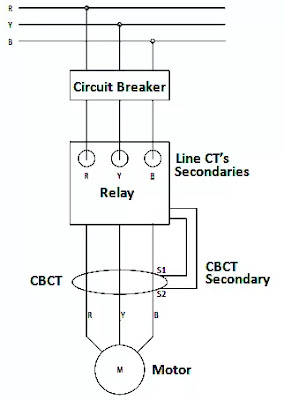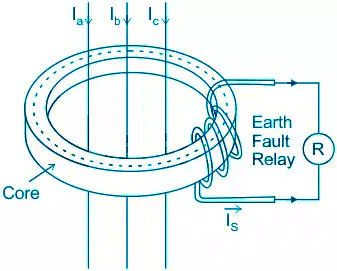## \$type=carousel\$clm=4

Core balance CT (also known as zero sequence CT) is a ring type current transformer used for earth fault protection.

## What is Core balance current transformer (CBCT)?

Core balance current transformer (also termed as CBCT) is a ring type current transformer (CT) through the centre of which either three single core cables or a single three core cable of three phase system passes. That three core cable forms primary winding of CBCT.

In other words we can define as a zero sequence CT (ZCT) is also known as core balance CT (CBCT) is a special type of current transformer (CT) which detects the presence of zero sequence current during short circuit fault (such as LG fault) condition that causes unbalance in 3-phase system.

Core balance Current Transformer works on the concept of balancing zero sequence current in three phase system. That's why it is also known as zero sequence current transformer (ZCT). Core balance CT is generally used for earth fault protection (or earth leakage protection) for medium voltage electrical system. A typical CBCT is as shown in below figure.

Unlike current transformer (CT), primary winding of CBCT is formed with three core cable passing through centre of its iron core and secondary coil of CBCT  is also wounded on its ring type iron core, and it is connected with earth fault relay.

## Working principle of CBCT or ZCT

A core balance current transformer (CBCT) works on the principle of balancing zero sequence current in 3-phase system. Hence it is also called as zero sequence current transformer (ZCT). The basic principle of working of zero sequence current transformer is Kirchhoff’s current low: the sum of current at any node of electrical circuit will be equal to zero.

During the normal operation of three phase system as the vector sum of its phase current ( Īa + Īb + Īc = 0 ) is zero. Therefore no any residual zero sequence current will present in primary winding of CBCT. Therefore there will not be any flux developed in the Core of CBCT. Hence no current will flow in secondary circuit of CBCT.

Whereas in abnormal condition, when sum of phase current will not zero. Then due to unbalancing of phase current, a zero sequence current will flow in the secondary circuit of CBCT. Hence earth fault relay connected with secondary of CBCT, will get energized and it isolate the healthy system with the help of circuit breaker.

Let Īa , Īb and  Īc are line currents of three phase system and Φa Φb and Φc are corresponding components of magnetic flux developed in the core of zero sequence CT or CBCT. Assuming that CBCT is operating in linear region, then magnetic flux developed in core of CBCT will be directly proportional to its corresponding line current. Hence it can be written as:

Φa = kIa

Φb = kIb

Φc = kIc

Here (k) is proportionality constant. Since here all three phase currents produces corresponding magnetic flux in the same core of ZCT (same magnetic material). Hence same proportionality constant (k) is used in all phase fluxes.

Hence resultant magnetic flux in the core of ZCT or CBCT will be

Φr = k(Īa + Īb + Īc________________(1)

But as we all know that from concept of symmetrical components

a + Īb + Īc) = 3Ī0 = Īn________________(2)

Here Īn is neutral current and Ī0 is zero sequence current in current transformer. Hence from above equations (1) and (2), we can conclude as

Φr = k.Īn ______________________(3)

Now, let’s consider two cases

Case 1: During normal operation of three phase system

As we know that Īa + Īb + Īc = 0 _______(4)

Here by comparing equations (1) and (4), we got result as:

Net resultant flux (Φr = 0) is zero. It means no current will flow in secondary circuit of CBCT. Hence earth fault relay will not operate in normal healthy condition.

Case 2: During earth fault (say LG fault) in abnormal condition, phase current in three core cable passing through centre of current transformer will not balance. Hence a zero sequence current will flow in secondary circuit of ZCT. For example consider the case of single line to ground fault (LG fault).

If = 3Ia0 = In _____________(5)

Comparing equation (5) with equation (3) we got that the net magnetic flux in core of CBCT will not zero. It has some finite value that will induce current in secondary circuit of ZCT. Therefore those secondary current flows in earth fault protection relay and activate it. Because of this reason, a core balance CT (CBCT) is also called zero sequence CT (ZCT).

## Application of CBCT or ZCT

The core balance current transformer (CBCT) is mainly used for earth fault protection of electrical machines. One of its important application as earth fault protection of induction motor, that is discussed below in detail.

### CBCT for motor protection

In most of industries, CBCT is being used for earth leakage protection of industrial motor ( 3-phase induction motor ). In this protection scheme, the core of CBCT surrounds the power cables connected with 3-phase induction motor. The earthing leads from the cable-sheath to earth must be taken through the eye of ZCT core. A typical diagram is as shown in below figure.CBCT for motor protection

The core of CBCT is excited by the current (Īa + Īb + Īc + Īsheath + Īearth).

The effect of ( Īsheath + Īearth ) is cancelled when the earthing leads are taken through the eye of CBCT. Hence the protection scheme responds only for (Īa + Īb + Īc) current in induction motor.

## Advantages of CBCT

The main advantage of using CBCT as earth fault protection scheme is that in this protection scheme only one CT core is used instead of three core as in conventional system. Thus the magnetic flux required for production of particular secondary current is reduced to one third (1/3), which is biggest advantage as the overall sensitivity of protection system is increased.

[ ##eye## Theory and working of Star-Delta Starter]

Also the number of secondary turns of CT is not required according to rated current of cable because no secondary current flows in normal operating condition as the three phase system current are balanced. This allows to optimize the secondary turns according to the effective primary pickup current of CT.

## Features of ZCT or CBCT

These are very attractive features of zero sequence CT (ZCT) or Core balance current transformer (CBCT), these are mentioned below as:

• High sensitivity
• Good linearity
• Reliable operation
• Convenient and easy installation

## Selection of CBCT or ZCT

The appropriate zero sequence CT or CBCT is selected based on the following factors.

• Nominal CT ratio: this should be such that even in smallest ground fault the current should be sufficient to operate earth fault relay.

• Minimum primary ground leakage current

• Minimum excitation current required for relay operating voltage

• Knee point voltage

• Dimensions and internal diameter of CT (internal diameter depends on size of cable)BLOGGER: 4
1.Very good article.. thanks

2.Well explained...

3.Very good...

4.This explanation is only applicable for delta connections, as I understand. For unbalanced star 4-wire connections (and practically, almost all of them are unbalanced), Ia+Ib+Ic = In. So you'd also need the neutral wire going through the core-balance transformer.

Name

Electrical Basics,8,Electrical Machine,3,Electrical Q & A,2,Electronics,3,Instrumentation,3,Mathematics,3,Power Factor,3,Power System,8,
ltr
item
Electrical-Technology | All about Electrical Engineering: Core balance Current Transformer (CBCT) or zero sequence CT (ZCT)
Core balance Current Transformer (CBCT) or zero sequence CT (ZCT)
Core balance CT (also known as zero sequence CT) is a ring type current transformer used for earth fault protection.